o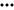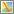OR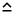Remember me Sign in
OR
Looking for an online, multi-user solution for your company?
Start here.
 Sign In Using: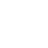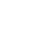Contact Us Sign In

Example: 5 Hours in 15 Days, or 11 Hours in 30 Days, in H60
Initialization script:
```	  var hrs15 = 0, hrs30 = 0;
```
Loop script:
```	  var icao = getfieldstr("ICAO Code");
if (strequal(icao, "H60"))
{
var ft = getfieldnum("Day Flight Time") +
getfieldnum("Night Flight Time");
hrs30 = hrs30 + ft;

if (getdaysago() <= 15)
hrs15 = hrs15 + ft;

if (hrs30 >= 11 || hrs15 >= 5)
currency_ok = 1;
}
```
How it works:
First, we get the ICAO code of the aircraft associated with the flight record; if it's "H60", we proceed with the rest of the logic. Next, we calculate the total flight time for the record and add it to `hrs30`. (We'll define the period for this currency definition as 30 days, so we know all records we see are within 30 days.)
Then, if `getdaysago()` returns 15 or less, we know the record is within the last 15 days, so we also add the flight time to `hrs15`.
Finally, we check to see if we've accumulated either 11 hours in `hrs30`, or 5 hours in `hrs15`. If either of these is true, our currency is valid.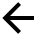Back to help index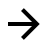Next article